Home | | Structural Dynamics and Earthquake Engineering | Codal provisions for seismic design

# Codal provisions for seismic design

1 International Building Code of USA 2000 2 New Zealand Standards NZS 1170.5 3 Eurocode 8 procedure (EC-8) 4 Uniform Building Code (UBC) 1997 5 National Building Code (NBC) of Canada (1995) 6 Mexican Federal District Code (MFDC) 1993 7 Japanese Society of Civil Engineers (JSCE) 2000

Codal provisions for seismic design

1  International Building Code of USA 2000

The base shear is given by

Vb = CsW                       --- 19.4

where W is the total weight and applicable portions of other loads and the seismic coefficient CS is given by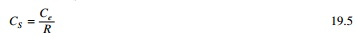This coefficient corresponding to R = 1 is known as the elastic seismic coefficient.

Ce = IC               --- 19.6

where I is the importance factor: I = 1 for most structures, I = 1.25 for structures that have substantial public hazard due to occupancy and I = 1.5 for essential facilities that are required for post earthquake recovery.

The period coefficient C depends on the location of the structures and site class.where T1 is the fundamental natural vibration period of the structure in secondswhere wi is the weight of the ith floor and ui are the floor displacements due to static application of a set of lateral loads Fi at floor levels i = 1, 2, 3,ŌĆ”,N. These forces Fi may be any reasonable distribution over the building height and need not be exactly the design lateral forces specified in the code.

The response modification factor R depends on several factors, including ductility capacity and inelastic performance of structural materials and systems during past earthquake. Specified values of R vary between 1.5 and 8.

The distribution of lateral force over the height of building is given by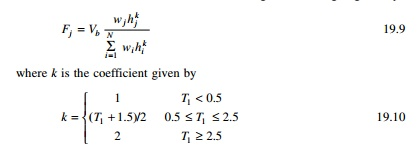The deterministic overturning moments are multiplied by a factor J. J = 1.0 for the top 10 storeys, 1ŌĆō0.8 for next ten storeys from top and varying linearly with the height 0.8 for remaining floors.

2  New Zealand Standards NZS 1170.5

Seismic design in New Zealand has evolved over the past 30 years from an ŌĆśallowable stress basisŌĆÖ to ŌĆśstrength capacityŌĆÖ approach. The concept of ŌĆśCapacity designŌĆÖ is well established as a way of thinking for New Zealand structural engineers and dominates their design approach. Over the past 15 years, the loading standards (NZS 4203-92, NZS 1170.5) have been used as a basis of design a ŌĆśconstant hazard design spectrumŌĆÖ. The spectra is not intended to be an ŌĆśearthquakeŌĆÖ spectrum but a spectrum for which the acceleration at each spectral period has an equal likelihood of being exceeded over some passage of time. New Zealand design spectra are anchored back to a constant hazard approach.

The forces acting on a structure as a result of ground shaking are usually determined by one of the following methods:

ŌĆó       Static analysis: using equivalent static force obtained from acceleration response spectra from horizontal earthquake motions.

ŌĆó       Dynamic analysis: either the modal response spectrum method or numerical integration time history method using earthquake records:

According to New Zealand standard for general structural design loading for buildings the equivalent static method of analysis can be applied only where at least one of the following criteria is satisfied:

ŌĆó       The height between the base and top of the structure does not exceed 15 m.

ŌĆó       The calculated fundamental period of vibration of the structure does not exceed 0.45 s.

ŌĆó       The structure satisfies the horizontal and vertical regularities requirement standard and has a fundamental period of vibration <2 s.

According to NZS 4203:1993[1.3] when the equivalent static force method is used, the design horizontal seismic forces acting at the base of the structure or at the serviceability limit state isCh(T1, ┬Ą) = basic seismic horizontal coefficient which depends on fundamental period of vibration T1, required structural ductility factor ┬Ą of the structure.

Ch(T1, 1) = basic seismic hazard coefficient when ┬Ą = 1. Sp ŌĆō structural performance factor, R ŌĆō risk factor, Z ŌĆō zone factor to take into account regional seismicity, Wt ŌĆō weight of structure and contents considered to be present during earthquake.

The horizontal seismic design force V given by Eqs 19.11 and 19.12 are distributed appropriately up to the height of the structure. ┬Ą is the structural ductility factor given by Ōłåmax/Ōłåy.

According to NZS 4203: 1992 (1.3) structural performance factor SP is taken as 2/3 unless specified otherwise in the material standard. A value of 2/3 is justified as a result of beneficial effects.

In some structures Sp Ōēł 1 may be more appropriate. The risk factor R varies between 1.3 for buildings dedicated to the preservation of human life or for which loss function would have a severe impact on society and 0.6 for buildings of secondary importance with a range of values between. The zone factor Z varies between 0.6 and 1.2. For most of the New Zealand zones, the value of Z corresponds to approximately to 5% damped spectral acceleration coefficient at a fundamental period of vibration of 0.2 s of 450 year return period uniform risk hazard spectra for elastic response. Figure 19.1 shows the response spectrum for the basic hazard acceleration coefficient for near or very stiff soil recommended by NZS-4203: 1992.

The above elastic response spectra for ultimate limit state have an assured return period of 450 years (approximately 10% of probability of exceedence in 50 years). NZS 3101: 1995[1.5] specifies values for displacement factors and design procedures for various categories of ductility of reinforces concrete (RC) structures.

3  Eurocode 8 procedure (EC-8)

The method is referred to as ŌĆśsimplified modal response spectrum analysisŌĆÖ rather than ŌĆśequivalent static analysisŌĆÖ and is restricted to structures that are not significantly affected by higher modes and/or stiffness irregularities. The base shear is calculated as

VB = Sd(T1)W                          --- --19.13

Sd(T1) is the ordinate of the design spectrum corresponding to fundamental period T1 of the structure and W is the gravity load contributing W.

The inertia forces are taken as permanent loads G and portion ŽłEQ of variable live loading Q. The fundamental period T1 can be estimated for a proper eigen value analysis or from empirical formula included in the code.

The lateral force corresponding to VB can be calculated as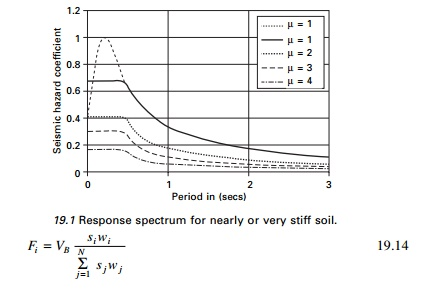where Fi is the horizontal force acting in storey i, si and sj are displacement of masses mi, mj in the fundamental mode shape. The code assumes the fundamental mode shape is increasing linearly with the height of the building, hence si is substituted for zi.

In order to cover uncertainties in the distribution of mass and stiffness as well as spatial variability of ground motion, an accidental eccentricity of the loads Fi with respect to mass centre CM of the storey has to be introduced in the analysis and is equal to

e1i = ┬▒0.05Li                   19.15

where Li is the floor dimension perpendicular to the direction of force Fi. The eccentricity e1 is additional to any existing eccentricity e0 between the stiffness centre CS and mass centre CM at any storey. Hence torsional moment Mt = Fi(e0 + e1) or simply Fie1 if a three-dimensional model is used to act at the mass centre. The load combination including seismic loading isŌĆś+ŌĆÖ means to be combined with; G = permanent dead load; Q = variable imposed load; ╬│1 important factor; Ed design value for seismic action.

The criterion for the required number of modes to be included in the analysis is two-fold:

1.     The sum of effective modal mass should amount to at least 90% of the total mass.

2.     All modes with effective mass >5% of the total mass should be considered.

The modal action should be combined with SRSS unless the period of two of them considered modes differ by less than 10%, in which case CQC approach should be used.

4  Uniform Building Code (UBC) 1997

The method is applicable to all buildings in the low seismicity zone (zone 1 and usual structures in seismic zone 2), regular structures up to 73 m and irregular structure having no more than five stories. The design base shear is given byW = seismic dead load, Ca, Cv I and R define the design spectrum. The seismic coefficients Ca, Cv of 1997 UBC are given in Table 19.2.

The load factor in the above equations should be increased by 10% for design of RC and masonry structures. There are two differences in the modal analysis procedure specified in UBC:

1.     The elastic rather than the design response spectrum is used for estimating actual effect.

2.     The elastic force calculated above is then scaled down to account for inelastic effects. This is done by adjusting them to 90% of VB used in the equivalent stated analysis in the case of regular structures 100% in case of irregular structures.

5  National Building Code (NBC) of Canada (1995)

The base shear is expressed as

VB = CsW                         -----19.22

The seismic coefficient Cs is given byU = 0.6 is a calibration factor applied to maintain the design base shear at the same level of protection (as in the preceding edition of the code) for buildings with good to excellent capability of resisting seismic loads. The elastic seismic coefficient is given by1.     Zonal velocity factor ╬│ varies between 0 for least seismic zone to 0.4 and the worst seismic zone.

2.     I = 1.5, 1.3 and 1 for post-disaster building, for schools and for other buildings respectively.

3.     F = 1.0, 1.3, 1.5, 2.0 foundation factors depending on soil category.

4.     S = seismic response factor varies with T1

For T1 < 0.5 sZa, Zv represent acceleration-related seismic zones and velocity-related seismic zones respectively. Canada is divided into seven zones based on each of the two criteria.

The empirical formula for calculating T1 is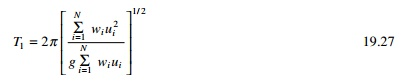where ui is the floor displacement of ith floor, wi is a set of lateral loads at floor levels. The elastic seismic coefficient Ce depends on pseudo-acceleration design spectrum scaled to ground velocity of 0.4 m/s (╬│ = 0.4).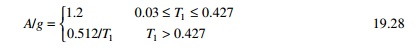The force modification factor R varies from 1 for brittle structure to 4 for ductile moment resisting space frames.

The distribution of lateral forces over the height of the building is determined fromThe National Building Code of Canada (NBC) was revised in 2005. The seismic hazard map is given and the seismic hazard is expressed as the most powerful ground motion that is likely to occur in an area for a given probability level. Building design for various earthquake loads is addressed in Sections 4.1.8, 9.20.1.2, 9.23.10.2 and 9.31.6.2 of the 2005 NBC. The seismic hazard values are described by spectral acceleration values at periods 0.2, 0.5, 1.0 and 2.0 s. It is a better measure of potential damage than the peak measure used by 1995 and thus improves earthquake design. PGA is still used in foundation design. The probability used in the 2005 NBC is 0.000404 per annum equivalent to 2% probability exceeding over 50 years. A building designed to tolerate a sideward pushing force equivalent to 40% of it own weight should prove earthquake-resistant.

6  Mexican Federal District Code (MFDC) 1993

The seismic coefficient is calculated as

Cs = Ce/QŌĆ▓                                   ---19.33

Elastic seismic coefficientT1 Fundamental time period, Tb and Tc = beginning and end of constant acceleration regions, Am, ╬Į, Tb, Tc are given in Table 19.3.

The elastic seismic coefficientOverturning moments determined are multiplied by reduction factor that varies linearly from 1.0 at the top of the building to 0.8 at its base to obtain design values.7  Japanese Society of Civil Engineers (JSCE) 2000

All codes except the JSCE code basically apply the concepts of the capacity design philosophy. Although the JSCE code does not follow the capacity design principles, it should be noted that basic ideas of seismic design are essentially similar and the JSCE code does not prescribe any specific design earthquake motion. The seismic code of buildings in Japan was revised in June 2000 to implement a performance-based structural engineering framework. The code provides the performance objectives, life safety and damage limitation of a building at the two corresponding levels of earthquake.

Design response spectra at engineering bedrock

The earthquake ground motion used for the seismic design at the life safety limit is the site-specific motion of an extremely rare earthquake which is expected to occur once in approximately 500 years. The engineering bedrock is assumed to be the soil layer whose shear wave velocity is >400 m/s. The basic design earthquake acceleration response spectra S0 of the seismic ground motion at the exposed outcrop engineering bedrock is given aswhere S0 = basic design acceleration response spectra in m/s2 and T = natural period. The level of earthquake ground motion used for the seismic design at the damage limit should be reduced to one-fifth of life safety.

Design response spectra at ground motion

Sa(T) = Gs(T) Z S0(T)           ---19.40

Where

Sa = design acceleration response spectra at ground surface m/s2, Gs = surface soil layer amplification factor, Z = seismic zone factor 0.7 to 1.0 and T = natural period.Gs1 = Gs ratio at T1

Gs2 = Gs ratio at T2

T1 = predominant period of surface soil layer for first mode T2 = predominant period of surface soil layer for second mode Minimum values of Gs8  Iranian code

Almost everywhere in Iran is prone to earthquake as two major earthquake belts run through the country. Every decade or so a major earthquake strikes Iran, resulting in many fatalities and collapsed buildings. Traditional Iranian buildings, especially in the rural areas, have very little resistance to earthquakes of higher magnitude.

After numerous major earthquakes, in particular that of 1963 in Bouein Zahra, the Iranian government began the preparation of code of practice for earthquake protection in 1967. IranŌĆÖs Ministry of Housing first published a code of practice for earthquake-resistant construction which requires buildings taller than 11 m to be made of RC or steel frames.

In 1993 the Iranian Building Research Centre further revised the code and after three stages of research, construction and design and the updated and revised Iranian code for seismic resistant design was published in 1997 (IS2800). This code was revised in 1999 and covers seismic design of RC and steel and masonry construction. According to IS2800 (1999) chapter 2, the seismic base shear coefficient is obtained fromwhere

V = base shear

w = total weight of the building (DL+0.2LL)

C = base shear coefficient

A = design base acceleration or ratio to gravity which may be 0.2, 0.25, 0.3, 0.35 depending on regions (0.3g for Bamm in region 2 of the seismic micro-organization map of Iran)

B = building response factor obtained from design response spectrum (amplification factor)

I = importance factor of the building = 0.8, 1.0 or 1.2 T = natural period of the building

T0 = corner period of the acceleration response spectrum dependent on soil type (0.4 0.5 0.7 1.0) 0.5 s for soil type in Bamm

R = building factor varying from 4 to 11 (for example, 4 for simple masonry with frame 6 for concentric steel-braced buildings)

H = height of the building from base in m

For a two storey masonry building B = 2.5, I = 1, R = 4, the base shear coefficient C is estimated as 0.19. Seismic lateral forces may be calculated from9  Chinese code

Before 1964 there was no seismic-resistant design code for buildings and other structures in China. Earthquake-resistant design was not considered for most buildings. A draft of seismic-resistant design code in China was prepared in 1964. The first official seismic code of China was issued in 1974. In 1975 and 1976 China suffered two strong earthquakes: 1975 earthquake of Haichung with a magnitude of 7.3 and Tangshan with a magnitude of 7.8. These two earthquakes were considered to be catastrophic disasters, killing 242 829 people. The code was revised and put into effect in 1993. Equivalent lateral force method was recommended.

The total horizontal seismic action FEK (base shear) is given bywhere weq is the total equivalent seismic weight of a building and ╬▒ is the seismic coefficient which can be determined fromwhere Tg is the characteristic period of vibration of the soil given in Table 19.4

The total seismic weight should be used when the structure is modelled as a single-degree-of-freedom (SDOF) system, 85% of total seismic weight if it is modelled as a multiple-degrees-of-freedom (MDOF) system. Table 19.5 gives values of ╬▒max.

The horizontal seismic force Fi applied at any level I of the building is given by

where ╬┤N is called additional seismic action coefficient given in Table 19.6. The overturning moment is given asFor any other country code one may refer to the international handbook of earthquake engineering codes by Maria Paz (1994).

10Indian Seismic Code 1893 ŌĆō Part 1 ŌĆō 2002

The first Indian Seismic Code (IS 1893) was first published in 1962 and it has since been revised in 1966, 1970, 1975 and 1984. More recently it was decided to split this code into a number of parts and Part 1 of the code containing general provisions (applicable to all structures) and specific provisions for buildings has been published. Some extracts of the code are given below.

The design horizontal seismic coefficient Ah for a structure can be determined fromProvided that for any structure with T Ōēż 0.1 s the value of Ah will not be less than Z/2 whatever may be the value of I/R.

Z = zone factor as given in Table 19.7 and it is for a maximum considered earthquake (MCE) and service life of structure in a zone. The factor 2 is used in the denominator to reduce MCE to design basis earthquake (DBE). I = importance factor depending upon the functional use of the structure characterized by hazardous consequences of its failure varying from 1 to 1.5. R = response reduction factor depending on the perceived seismic damage performance of the structure characterized y ductile or brittle deformations. However, the ratio of I/R Ōēż 1.

Sa/g ŌĆō average response acceleration coefficient for rock or soil sites given by for 5% damping. For rocky or hard soilThe response spectrum for IS1893-2002 is shown in Fig. 19.2 and the zone factors are shown in Table 19.7.

Table 19.8 gives the multiplying factors for obtaining spectral values for various other damping ratios.The seismic weight of each floor is its full dead load plus an appropriate amount of imposed load. The total design lateral force or design seismic base shear is given byDynamic analysis shall be performed to obtain the design seismic force and its distribution to different levels along the height of the building.

’éĢ   Regular building >40 m in the zone IV and V and >90 m in the height in      Zones II and III

’éĢ   Irregular building. All framed buildings >12 m in zones IV and V >40 m     in Zones II and III.

Dynamic analysis may be performed either by the time history method or the response spectrum method. However, base shear VB is compared with VB obtained by using approximate method. If VB < VB all the response quantities to be multiplied by VB /VB.Modal mass is calculated asThe peak storey shear force Vi in each first storey due to all modes considered is obtained by combining those due to each mode in accordance with square root of sum of squares (SRSS) or complete quadratic combination (CQC) rules.

Program 19.1: MATLAB program for IS1893 code

A program in MATLAB is written to calculate the shear in each storey as well as drift calculations for a multi-storey frame. Example 19.8 is solved by the MATLAB program and the results are also given below.

% IS1893 - 2002, Part 1 multistorey buildings % calculation of shear in each storey and drifts nst=6;

m=zeros(nst,nst);

K=zeros(nst,nst);

ma=zeros(nst,1); ak=zeros(nst,1);

t=zeros(nst,1);

sa=zeros(nst,1);

pf=zeros(nst,1);

d=zeros(nst,nst);

v=zeros(nst,nst);

vv=zeros(nst,nst);

vr=zeros(nst,1);

ah=zeros(nst,1);

sf=zeros(nst,nst);

% ***************************************************

%input data %*****************************************************

%give masses for various floors starting from ground

ma(1,1)=262.59e3;ma(2,1)=262.59e3;ma(3,1)=262.59e3;ma(4,1)=262.59e3;

ma(5,1)=262.59e3;ma(6,1)=229.934e3;

%give stiffnesses for various floors starting from ground

ak(1,1)=586926e3;ak(2,1)=586926e3;ak(3,1)=586926e3;ak(4,1)=318652e3;

ak(5,1)=318562e3;ak(6,1)=318562e3;

%soil s=1 for rocky soil s=2 medium soil s=3 for soft soil

s=1;

%give zone number

zo=4;

% give damping % for concrete 5% for steel 2% etc

da=5;

% importance of the structure

is=1;

% response reduction factor

r=3;

% height of the building

ht=21;

% width of the building

width=14;

%it=1 for rc frame buildig without brick infil panel

%it =2 for steel frame building with out brick infil panel

%it=3 for all buildings with brick infill panel

it=3;

% *******************************************************

% input completed

%********************************************************

if zo==2

z=0.1;

end

if zo==3

z=0.16;

end

if zo==4 z=0.24;

end

if zo==5 z=0.36;

end fid=fopen(ŌĆśoutput.tableŌĆÖ,ŌĆśwŌĆÖ); fprintf(fid,ŌĆśzone = %2i\nŌĆÖ,zo); fprintf(fid,ŌĆśsoil=%2i\nŌĆÖ,s);

fprintf(fid,ŌĆśimportance factor=%2i\nŌĆÖ,is);

fprintf(fid,ŌĆśresponse reduction factor=%2i\nŌĆÖ,r); fprintf(fid, ŌĆśstorey mass\nŌĆÖ);

for i=1:nst

fprintf(fid,ŌĆś%2i , %f\nŌĆÖ,i,ma(i,1)); end

fprintf(fid,ŌĆś storey stiffness\nŌĆÖ); for i=1:nst

fprintf(fid,ŌĆś%2i,%f\nŌĆÖ,i,ak(i,1)); end

ak(nst+1,1)=0.0; nstm1=nst-1; weight=0.0;

for i=1:nstm1 ip1=i+1; m(i,i)=ma(i,1);

K(i,i)=ak(i,1)+ak(ip1,1); K(i,ip1)=-ak(ip1,1); weight=weight+m(i,i)*9.81;

end K

m(nst,nst)=ma(nst,1);

K(nst,nst)=ak(nst,1);

weight=weight+ma(nst,1)*9.81; for i=1:nst

for j=i:nst K(j,i)=K(i,j);

end end m

K ki=inv(K); kim=ki*m;

[v,d]=eig(kim); for i=1:nst

for j=1:nst v(i,j)=v(i,j)/v(nst,j);

end end

for i=1:nst om(i,1)=1/sqrt(d(i,i)); t(i,1)=2*pi/om(i,1);

end

fprintf(fid,ŌĆśmode period\nŌĆÖ);

for i=1:nst fprintf(fid,ŌĆś%2i,%f\nŌĆÖ,i,t(i,1));

end

for j=1:nst

fprintf(fid,ŌĆśmode shape for mode = %2i\nŌĆÖ,j); for i=1:nst

fprintf(fid,ŌĆś%f\nŌĆÖ,v(i,j)); end

end if s<2

ml=0.4;

co=1; elseif (s>2)

ml=0.67;

co=1.67; else

ml=0.55;

co=1.36; end

for i=1:nst

if t(i,1)<0.1 sa(i,1)=1+15*t(i,1); elseif t(i,1)>ml

sa(i,1)=co/t(i,1);

else

sa(i,1)=2.5;

end end

if da==0 mf=3.2;

end

if da==2; mf=1.4;

end

if da==5; mf=1.0;

end

if da==7; mf=0.9;

end

if da==10; mf=0.8;

end

fprintf(fid,ŌĆś sa/g \nŌĆÖ); for i=1:nst

sa(i,1)=mf*sa(i,1);

fprintf(fid,ŌĆś%f\nŌĆÖ,sa(i,1)); end

for i=1:nst sum=0.0; sum1=0.0; for j=1:nst

sum=sum+m(j,j)*v(j,i);

sum1=sum1+m(j,j)*v(j,i)^2; end

%sum

%sum1

pf(i,1)=sum/sum1;

pmas(i,1)=pf(i,1)*sum*9.81/weight; end

fprintf(fid,ŌĆśmode participation factor\nŌĆÖ); for i=1:nst

fprintf(fid,ŌĆś%2i, %f\nŌĆÖ,i,pf(i,1)); end

fprintf(fid,ŌĆś percentage of modal masses\nŌĆÖ); for i=1:nst

fprintf(fid,ŌĆś%2i, %f\nŌĆÖ,i,pmas(i,1)); end

for i=1:nst ah(i,1)=z*is*sa(i,1)/(2.0*r);

if t(i,1)<0.1 & ah(i,1) <z/2 ah(i,1)=z/2;

end end

fprintf(fid,ŌĆśmode Ah\nŌĆÖ); for i=1:nst

fprintf(fid,ŌĆś%2i , %f\nŌĆÖ,i,ah(i,1)); end

for i=1:nst for j=1:nst

sf(j,i)=pf(i,1)*ah(i,1)*m(j,j)*v(j,i); end

end %sf

for j=1,nst vv(nst+1,j)=0.0;

end

for j=1:nst for i=1:nst

ii=nst-i+1; vv(ii,j)=sf(ii,j)+vv(ii+1,j);

end end

for i=1:nst for j=1:nst

vv(i,j)=9.81*vv(i,j); end

end

fprintf(fid,ŌĆÖ shear in various stories for various modes\nŌĆÖ); for i=1:nst

fprintf(fid,ŌĆÖ for mode =%2i\nŌĆÖ,i); for j=1:nst

fprintf(fid,ŌĆÖ%2i %f\nŌĆÖ,j,vv(j,i)); end

end

for i=1:nst vr(i,1)=0.0; for j=1:nst

vr(i,1)=vr(i,1)+vv(i,j)^2; end

vr(i,1)=sqrt(vr(i,1)); end

fprintf(fid,ŌĆś base shear as per modal calculation\nŌĆÖ); fprintf(fid,ŌĆś%f\nŌĆÖ,vr(1,1));

fprintf(fid,ŌĆś approximate calculation as per the code\nŌĆÖ); if it==1

tn=0.075*ht^0.75; end

if it==2 tn=0.085*ht^0.75;

end

if it==3 tn=0.09*ht/sqrt(width);

end

fprintf(fid,ŌĆś fundamental natural period = %f\nŌĆÖ,tn); if tn<0.1

saf=1+15*tn; elseif tn>ml saf=co/tn;

else saf=2.5;

end ahf=saf*z*is/(2*r); if tn<0.1 & ahf <z/2

ahf=z/2; end ahf=ahf*mf; weight vb=ahf*weight

fprintf(fid,ŌĆś base shear as per codal approximate period %f\nŌĆÖ,vb); factor=vb/vr(1,1);

if factor>1 for i=1:nst

vr(i,1)=factor*vr(i,1); end

end

fprintf(fid,ŌĆś resultant shear in various stories\nŌĆÖ); for i=1:nst

fprintf(fid,ŌĆś%2i %f\nŌĆÖ,i,vr(i,1)); end

fprintf(fid,ŌĆś drift in various stories\nŌĆÖ); for i=1:nst

dr(i,1)=vr(i,1)/ak(i,1); fprintf(fid,ŌĆś%2i %f \nŌĆÖ,i,dr(i,1));

end dr;

fclose(fid);

OUTPUT

zone = 4 soil= 1

importance factor= 1 response reduction factor= 3 storey mass 1,262590.000000 2,262590.000000 3,262590.000000 4,262590.000000 5,262590.000000 6,229934.000000

storey stiffness

1,586926000.000000

2,586926000.000000

3,586926000.000000

4,318652000.000000

5,318562000.000000

6,318562000.000000 mode period 1,0.589328 2,0.223705 3,0.138951 4,0.072667 5,0.104123 6,0.093019

mode shape for mode = 1 0.182786 0.356276 0.511647 0.749899 0.917955 1.000000

mode shape for mode = 2 ŌĆō0.581337 ŌĆō0.957496 ŌĆō0.995713 ŌĆō0.418807 0.430599 1.000000

mode shape for mode = 3 0.641094 0.695710 0.113885 ŌĆō1.149675 ŌĆō0.475857 1.000000

mode shape for mode = 4 ŌĆō83.585291 112.412194 ŌĆō67.595631 17.300351 ŌĆō4.396286 1.000000

mode shape for mode = 5 ŌĆō1.155218

ŌĆō0.428418

0.996338

0.630861

ŌĆō1.628296

1.000000

mode shape for mode = 6 3.061088 ŌĆō0.126548 ŌĆō3.055856 3.038515 ŌĆō2.293290 1.000000

sa/g

1.696847

2.500000

2.500000

2.090004

2.500000

2.395278

mode participation factor 1, 1.329829 2, -0.472949 3, 0.210335 4, -0.001019 5, -0.110181 6, 0.043985

percentage of modal masses 1, 0.813473 2, 0.132582 3, 0.025087 4, 0.004336 5, 0.013297 6, 0.011226

mode Ah 1,0.067874 2,0.100000 3,0.100000 4,0.120000 5,0.100000 6,0.120000

shear in various stories for various modes for mode = 1

1 835695.230296

2 793195.356194

3 710356.969254

4 591392.874440

5 417032.256536

6 203596.660163 for mode = 2

1 200671.454855

2 129845.975774

3 13192.337190

4 -108117.437477

5 -159141.610729

6 -106680.868269 for mode = 3

1 37970.806318

2 3234.813194

3 -34460.412555 4 -40630.981084 5 21661.132553 6 47444.203966 for mode = 4

1 7875.062753

2 -18466.077913

3 16959.597905

4 -4342.547766

5 1109.499737

6 -275.949577 for mode = 5

1 20126.010937

2 -12662.189159

3 -24821.840801 4 3456.897732 5 21362.427841 6 -24852.979108 for mode = 6

1 20389.068926

2 -21231.973689

3 -19511.317627 4 22038.591323 5 -19275.537688 6 11905.898799

base shear as per modal calculation 860802.021330

approximate calculation as per the code

fundamental natural period = 0.505124

base shear as per codal approximate period 1198572.993130 resultant shear in various stories

1 1198572.993130

2 1119971.883836

3 991684.516096

4 839604.037191

5 623536.713435

6 329037.218693 drift in various stories

1 0.002042

2 0.001908

3 0.001690

4 0.002635

5 0.001957

6 0.001033

Study Material, Lecturing Notes, Assignment, Reference, Wiki description explanation, brief detail
Civil : Structural dynamics of earthquake engineering : Codal provisions for seismic design |